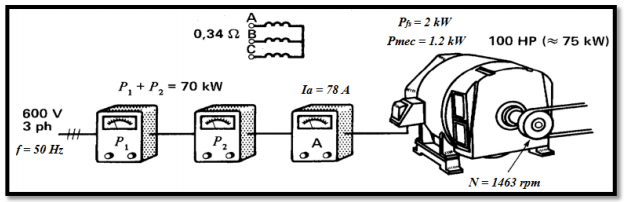# Study-of-induction motor-4

EXERCISE : STUDY OF AN INDUCTION MOTOR

A 600V line powers a three-phase induction motor with a nominal power of 100 hp (≈ 75 kW). Two wattmeters placed in the line wires indicate a total power of Pa = 70kW and an amperemeter indicates a line current of  Ia = 78A. Accurate measurements indicate a speed of rotation of  N = 1463 rpm. In addition, the following information is provided:
– The iron losses in the stator: Pfs = 2kW
– Ventilation and mechanical losses: Pmec = 1.2kw
– Resistance between two stator terminals : 2×Rs = 0.34ΩWe suppose STAR connexion.
1- Give the number of poles of machine stator and the slip s of the machine
2- Calculate the stator joule losses Pjs
3- Calculate the electromechanical power delivered to the rotor Pem
4- Deduce the joule losses in the rotor Pjr
5- Calculate the useful torque Tu of the motor
6- Calculate the efficiency of the motor η

CORRECTION

1)N= 1463rpm →Ns = 1500rpm →4 poles(synchronism speed: 1500rpm).

S = (Ns – N)/Ns = (1500 -1463)/1500  = 2.4%

2) Stator Joule losses : Pjs = 3RsIa2 = 3×0.34/2 ×782= 3.1kW

3) Pem = Pa – (Pjs + Pfs) = 70 – 3.1 – 2 = 64.9kW

4) Rotor Joule losses: Pjr = s×Pem = 0.024×64.9 = 1.5kW

5) Tu = Pun = (64900 – 1300 -1200)/70000 = 407.3Nm

6) η = Pu/Pa = (64900 – 1300 -1200)/70000 = 89%